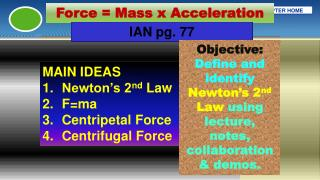DownloadDownload PresentationForces

Forces

Download PresentationForces

- - - - - - - - - - - - - - - - - - - - - - - - - - - E N D - - - - - - - - - - - - - - - - - - - - - - - - - - -
Presentation Transcript

1. Force = Mass x Acceleration Forces IAN pg. 77 Objective: Define and identify Newton’s 2nd Law using lecture, notes, collaboration& demos. • MAIN IDEAS • Newton’s 2nd Law • F=ma • Centripetal Force • Centrifugal Force

2. I. Newton’s 2nd Law A. Newton realized that inertia depends on mass and the amount of force exerted on an object. B. Mass and force form Newton’s Second Law. 1. Its just as hard to get something to move as it is to stop it from moving.

3. C. Newton’s 2nd Law of Motion An objects acceleration depends on force that acts upon it and the mass of the object.

4. Newton’s 2nd Law Top ½ IAN pg. 76 F = Force m = Mass a = Acceleration

5. II. Formula for Force A. Newton’s 2nd Law can be expressed by: F = m x a m= F / a a = F / m F m a

6. B. Force is measured in Newtons(N) C. Mass is in (kg) Kilograms or (g) grams D. Acceleration is in (m/s/s) E. The force of Earth’s gravity is 9.8 m/s/s

7. A boy accelerates a toy car with a mass of 1 kilogram with an acceleration rate of 3 m/s/s. What is the force that is exerted? Ex. F = m x a Force = 1 kg X 3 m/s/s Force = 3 N of force

8. A tennis ball with a mass of .2 kg is dropped from the balcony. At what force is the ball moving as it falls? Ex. F = m x a Force = .2 kg X 9.8 m/s/s Force = 1.96 N of force OR 2.0 N of force

9. How much more force needed to make the 2nd Car accelerate at the same rate?

10. III. Circular Motion A. Objects move in a straight path, unless a force acts on it. 1. When a car turns, you will continue to move straight ahead according to Newton’s First Law. Think About it!! All object act according to Newton 1st Law

11. 2. All objects want to travel in a straight line Newton’s 1st Law/Inertia B. Centripetal force is the inward force that keeps objects moving in a circular path.

12. C. Centrifugal Force – outward equal opposite force of centripetal due to inertia (Not an exerting force!) Exerting Force

13. 1. The Path of Inertia – relates to Newton’s 1st Law. Newton’s 1st Law Not an Exerting Force Exerting Force